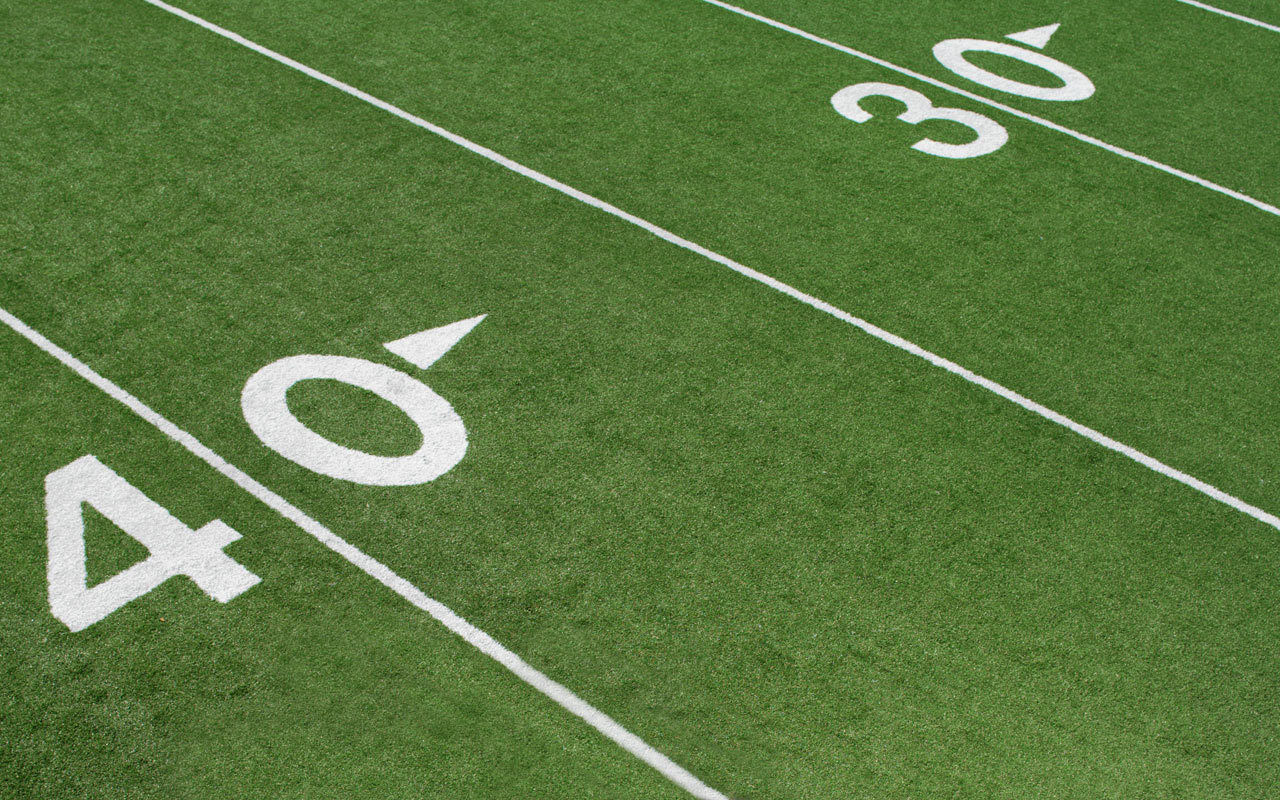top of pageStars Who Participated in College Athletics

Jaden Seier (2022)= Concordia University (NE) (Football). Position= LB

Aaron O'Brien(2022)= Nebraska Wesleyan (Football). Position= LB

Heinrinch Haarberg (2021)= University of Nebraska-Lincoln (Football). Position= QB

Logan O'Brien (2021)= Chadron State College (Football). Position= LB

Samson David (2021)= University of Nebraska-Kearney (Football). Position= DB

Spencer Hogeland (2021)= University of Nebraska-Kearney (Football). Position= K

Seth Moore (2021)= Concordia University (NE) (Football). Position DL

Eli Richter (2019)= University of Nebraska-Lincoln (Football). Position= OL/DL

Linden Howe (2019)= Drake University (Football). Position= LB

Sam Clinch (2019)= University of Nebraska-Kearney (Football). Postion= OL

Jason Seitz (2018)= Augustana University (Football). Postion= WR

Alex O'Brien (2018)= Nebraska Wesleyan (Football). Position= WR

Caleb Hoyt (2018)= University of Nebraska-Kearney (Football). Position= K

Jake Gappa (2018)= University of Nebraska-Kearney (Football). Position= LB

Brett Kaiser (2018)= University of Nebraska-Kearney (Football). Position= WR

Cole Staab (2018)= Hastings College (Baseball). Position= Outfielder

Eric Pacheco (2017)= Buena Vista University (Football). Position= WR

Nathan Williams (2017)= Doane University (Football). Position= LB

Creighton Hamik (2016)= Univeristy of Nebraska-Lincoln (Football). Position= LB {UNK 2017}

Grant Buschkoetter (2016)= University of Nebraska-Kearney (Football). Position= K

Ben Palmer (2016)= Rochester Institute of Technology (Rugby). Position= Wing

Nick Brungardt (2015)= University of Nebraska-Kearney (Football). Position=OL

Dennis Cabanting (2015)= University of Nebraska-Kearney (Football). Position=DL

Chase Murphy (2015)=Dakota Weseleyan University (Football). Positions=K

Nathan Howe (2015)=Hastings College (Football). Position=LB

Blake Holtmeier (2014)=University of Nebraska-Lincoln (Football). Position=WR (UNK 2016)

Thomas Connely  (2014)=University of Nebraska-Lincoln (Football). Position=LB/S

Jack Graham (2014)=Northwest Missouri State (Football). Position=OLB

Abe Clinch (2013)=Fort Hays State University (KS)(Football). Position=QB

David Squiers (2013)=University of Nebraska-Kearney (Football). Position=OL

Alex Lowe (2013)=St. John's University (MN)(Football). Position=LB

Brett James (2013)=Concordia University (NE)(Football). Position=WR

Anthony Pacheco (2012)=University of Nebraska-Kearney (Baseball)

Keagan Fitzgerald (2012)=University of Nebraska-Kearney (Baseball) [1 year at Doane College]

Drew Bulter (2011)=University of Nebraska-Kearney (Baseball)

Creighton Pearse (2011)=Concordia Universtiy (NE) (Cross Country/Track)

Matt Bowman (2010)=University of Nebraska-Kearney(Football) [1 yr @Kansas State]. Position=DE

Jared Reinke (2010)=University of Nebraska-Kearney (Football). Position=FS

Kris Kaczanowski (2010)=Concordia Universtiy (NE) (Football). Position=RB

Michael Klinginsmith (2009)= University of Nebraska-Lincoln (Wrestling)

Brandon Pacheco (2009)= University of Nebraska-Kearney (Baseball)

Darius Smith (2008)=Concordia University(NE)(Football). Position=RB

Jake Altmaier (2006)=University of Nebraska-Kearney (Football). Position=QB

Blake Cover (2005)=University of Nebraska-Kearney (Baseball). Creighton University (1 year)

Rick Bazata (2005)= Doane College (Football). Position= K

Brice Wiltgen (2004)=University of Nebraska-Kearney (Football). Position=FS

Tyson Compton (2004)=Doane College (NE)(Football). Position=QB

Tyler Steffesnmeier (2004)=Nebraska Weslyan (Football). Position=DB

Drew Sorensen (2004)= Hastings College (Wrestling)

Andy Butler (2003)=Hastings College (Football). Position=OL

Scott Bazata (2003)=Doane College (NE) (Soccer & Golf)

Jasen Nickman (2002)=University of Nebraska-Omaha (Football). Position=DB

Shawn Donahue (2001)=University of Nebraska-Kearney( Football). Position=WR

Shane Killion (2001)=University of Nebraska-Kearney (Football). Position=DL

Chad Ledbetter (1999)= Wayne State College (Football). Position= OL

Mitch Ebner (1999)= Wayne State College (Football). Position= WR

Gabe Compton (1991)=William-Jewell College (Football)

bottom of page